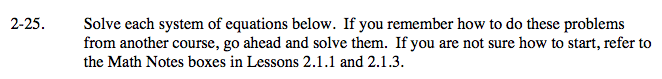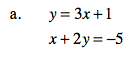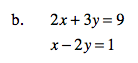### Home > CAAC > Chapter 14 > Lesson 14.2.1.2 > Problem2-25

2-25.Substitute 3x + 1 for y in the second equation.

x + 2(3x + 1) = −5

(−1, −2)Multiply the entire second equation by −2.

−2x + 4y = −2